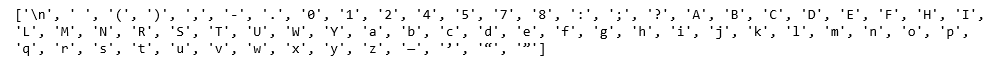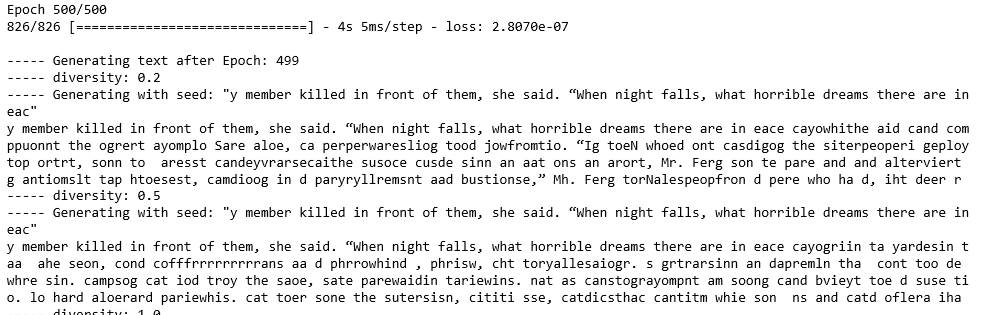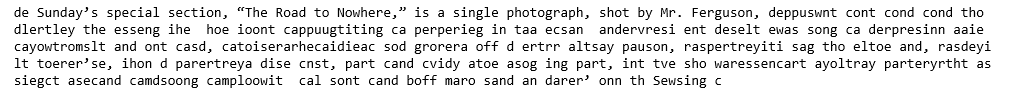# Text Generation using Recurrent Long Short Term Memory Network

This article will demonstrate how to build a Text Generator by building a Recurrent Long Short Term Memory Network. The conceptual procedure of training the network is to first feed the network a mapping of each character present in the text on which the network is training to a unique number. Each character is then hot-encoded into a vector which is the required format for the network.

The data for the described procedure was downloaded from Kaggle. This dataset contains the articles published in the New York Times from April 2017 to April 2018. seperated according to the month of publication. The dataset is in the form of .csv file which contains the url of the published article along with other details. Any one random url was chosen for the training process and then on visiting this url, the text was copied into a text file and this text file was used for the training process.

Step 1: Importing the required libraries

 `from` `__future__ ``import` `absolute_import, division, ` `                       ``print_function, unicode_literals ` ` `  `import` `numpy as np ` `import` `tensorflow as tf ` ` `  `from` `keras.models ``import` `Sequential ` `from` `keras.layers ``import` `Dense, Activation ` `from` `keras.layers ``import` `LSTM ` ` `  `from` `keras.optimizers ``import` `RMSprop ` ` `  `from` `keras.callbacks ``import` `LambdaCallback ` `from` `keras.callbacks ``import` `ModelCheckpoint ` `from` `keras.callbacks ``import` `ReduceLROnPlateau ` `import` `random ` `import` `sys `

 `# Changing the working location to the location of the text file ` `cd C:\Users\Dev\Desktop\Kaggle\New York Times ` ` `  `# Reading the text file into a string ` `with ``open``(``'article1.txt'``, ``'r'``) as ``file``: ` `    ``text ``=` `file``.read() ` ` `  `# A preview of the text file     ` `print``(text) `Step 3: Creating a mapping from each unique character in the text to a unique number

 `# Storing all the unique characters present in the text ` `vocabulary ``=` `sorted``(``list``(``set``(text))) ` ` `  `# Creating dictionaries to map each character to an index ` `char_to_indices ``=` `dict``((c, i) ``for` `i, c ``in` `enumerate``(vocabulary)) ` `indices_to_char ``=` `dict``((i, c) ``for` `i, c ``in` `enumerate``(vocabulary)) ` ` `  `print``(vocabulary) `Step 4: Pre-processing the data

 `# Dividing the text into subsequences of length max_length ` `# So that at each time step the next max_length characters  ` `# are fed into the network ` `max_length ``=` `100` `steps ``=` `5` `sentences ``=` `[] ` `next_chars ``=` `[] ` `for` `i ``in` `range``(``0``, ``len``(text) ``-` `max_length, steps): ` `    ``sentences.append(text[i: i ``+` `max_length]) ` `    ``next_chars.append(text[i ``+` `max_length]) ` `     `  `# Hot encoding each character into a boolean vector ` `X ``=` `np.zeros((``len``(sentences), max_length, ``len``(vocabulary)), dtype ``=` `np.``bool``) ` `y ``=` `np.zeros((``len``(sentences), ``len``(vocabulary)), dtype ``=` `np.``bool``) ` `for` `i, sentence ``in` `enumerate``(sentences): ` `    ``for` `t, char ``in` `enumerate``(sentence): ` `        ``X[i, t, char_to_indices[char]] ``=` `1` `    ``y[i, char_to_indices[next_chars[i]]] ``=` `1`

Step 5: Building the LSTM network

 `# Building the LSTM network for the task ` `model ``=` `Sequential() ` `model.add(LSTM(``128``, input_shape ``=``(max_length, ``len``(vocabulary)))) ` `model.add(Dense(``len``(vocabulary))) ` `model.add(Activation(``'softmax'``)) ` `optimizer ``=` `RMSprop(lr ``=` `0.01``) ` `model.``compile``(loss ``=``'categorical_crossentropy'``, optimizer ``=` `optimizer) `

Step 6: Defining some helper functions which will be used during the training of the network

Note that the first two functions given below have been referred from the documentation of the official text generation example from the Keras team.

a) Helper function to sample the next character:

 `# Helper function to sample an index from a probability array ` `def` `sample_index(preds, temperature ``=` `1.0``): ` `    ``preds ``=` `np.asarray(preds).astype(``'float64'``) ` `    ``preds ``=` `np.log(preds) ``/` `temperature ` `    ``exp_preds ``=` `np.exp(preds) ` `    ``preds ``=` `exp_preds ``/` `np.``sum``(exp_preds) ` `    ``probas ``=` `np.random.multinomial(``1``, preds, ``1``) ` `    ``return` `np.argmax(probas) `

b) Helper function to generate text after each epoch

 `# Helper function to generate text after the end of each epoch ` `def` `on_epoch_end(epoch, logs): ` `    ``print``() ` `    ``print``(``'----- Generating text after Epoch: % d'` `%` `epoch) ` ` `  `    ``start_index ``=` `random.randint(``0``, ``len``(text) ``-` `max_length ``-` `1``) ` `    ``for` `diversity ``in` `[``0.2``, ``0.5``, ``1.0``, ``1.2``]: ` `        ``print``(``'----- diversity:'``, diversity) ` ` `  `        ``generated ``=` `'' ` `        ``sentence ``=` `text[start_index: start_index ``+` `max_length] ` `        ``generated ``+``=` `sentence ` `        ``print``(``'----- Generating with seed: "'` `+` `sentence ``+` `'"'``) ` `        ``sys.stdout.write(generated) ` ` `  `        ``for` `i ``in` `range``(``400``): ` `            ``x_pred ``=` `np.zeros((``1``, max_length, ``len``(vocabulary))) ` `            ``for` `t, char ``in` `enumerate``(sentence): ` `                ``x_pred[``0``, t, char_to_indices[char]] ``=` `1.` ` `  `            ``preds ``=` `model.predict(x_pred, verbose ``=` `0``)[``0``] ` `            ``next_index ``=` `sample_index(preds, diversity) ` `            ``next_char ``=` `indices_to_char[next_index] ` ` `  `            ``generated ``+``=` `next_char ` `            ``sentence ``=` `sentence[``1``:] ``+` `next_char ` ` `  `            ``sys.stdout.write(next_char) ` `            ``sys.stdout.flush() ` `        ``print``() ` `print_callback ``=` `LambdaCallback(on_epoch_end ``=` `on_epoch_end) `

c) Helper function to save the model after each epoch in which loss decreases

 `# Defining a helper function to save the model after each epoch ` `# in which the loss decreases ` `filepath ``=` `"weights.hdf5"` `checkpoint ``=` `ModelCheckpoint(filepath, monitor ``=``'loss'``, ` `                             ``verbose ``=` `1``, save_best_only ``=` `True``, ` `                             ``mode ``=``'min'``) `

d) Helper function to reduce the learning rate each time the learning plateaus

 `# Defining a helper function to reduce the learning rate each time ` `# the learning plateaus ` `reduce_alpha ``=` `ReduceLROnPlateau(monitor ``=``'loss'``, factor ``=` `0.2``, ` `                              ``patience ``=` `1``, min_lr ``=` `0.001``) ` `callbacks ``=` `[print_callback, checkpoint, reduce_alpha] `

Step 7: Training the LSTM model

 `# Training the LSTM model ` `model.fit(X, y, batch_size ``=` `128``, epochs ``=` `500``, callbacks ``=` `callbacks) `Step 8: Generating new and random text

 `# Defining a utility function to generate new and random text based on the ` `# network's learnings ` `def` `generate_text(length, diversity): ` `    ``# Get random starting text ` `    ``start_index ``=` `random.randint(``0``, ``len``(text) ``-` `max_length ``-` `1``) ` `    ``generated ``=` `'' ` `    ``sentence ``=` `text[start_index: start_index ``+` `max_length] ` `    ``generated ``+``=` `sentence ` `    ``for` `i ``in` `range``(length): ` `            ``x_pred ``=` `np.zeros((``1``, max_length, ``len``(vocabulary))) ` `            ``for` `t, char ``in` `enumerate``(sentence): ` `                ``x_pred[``0``, t, char_to_indices[char]] ``=` `1.` ` `  `            ``preds ``=` `model.predict(x_pred, verbose ``=` `0``)[``0``] ` `            ``next_index ``=` `sample_index(preds, diversity) ` `            ``next_char ``=` `indices_to_char[next_index] ` ` `  `            ``generated ``+``=` `next_char ` `            ``sentence ``=` `sentence[``1``:] ``+` `next_char ` `    ``return` `generated ` ` `  `print``(generate_text(``500``, ``0.2``)) `My Personal Notes arrow_drop_upCheck out this Author's contributed articles.

If you like GeeksforGeeks and would like to contribute, you can also write an article using contribute.geeksforgeeks.org or mail your article to contribute@geeksforgeeks.org. See your article appearing on the GeeksforGeeks main page and help other Geeks.

Please Improve this article if you find anything incorrect by clicking on the "Improve Article" button below.